LEGACY CONTENT. If you are looking for Voteview.com, PLEASE CLICK HERE

This site is an archived version of Voteview.com archived from University of Georgia on May 23, 2017. This point-in-time capture includes all files publicly linked on Voteview.com at that time. We provide access to this content as a service to ensure that past users of Voteview.com have access to historical files. This content will remain online until at least January 1st, 2018. UCLA provides no warranty or guarantee of access to these files.

45-733 Probability and Statistics I (3rd Mini AY 1999-2000 Flex-Mode and Flex-Time)

2.36 This is just like the men-women committee examples done in class. Clearly, there are [8 choose 4] outcomes. There are 3 undergraduates and 5 graduate students. Hence,
```
æ3öæ5ö
ç ÷ç ÷
è2øè2ø
P(E) = ---------
æ8ö
ç ÷
è4ø
```
2.37 Clearly, there are [52 choose 2] outcomes. There are 4 Aces and there are 12 Face cards (i.e., Jacks, Queens, Kings). Hence,
```
æ4öæ12ö
ç ÷ç  ÷
è1øè 1ø
P(E) = ---------
æ52ö
ç  ÷
è 2ø
```
2.38 The number of ways to divide the 9 motors between the three production lines is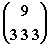. There are 2 motors from a particular supplier and 7 not from that supplier. Hence, there are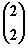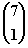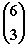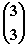=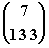ways to assign motors such that the 2 from the particular supplier go to the first line. Hence,

P(two to first line) =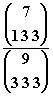2.51a. From Table P(A) = .4
1. From Table P(B) = .37
2. From Table P(A Ç B) = .10
3. Using parts a-c: P(A È B) = .40 + .37 -.10 = .67
4. P(Ac) = .6
5. [P(A È B)]c = .33
6. [P(A Ç B)]c = .9
7. P(A | B) = P(A Ç B)/P(B) = .1/.37
8. P(B | A) = P(A Ç B)/P(A) = .1/.4
2.65 Let A = Device gets past first inspector and B = device gets past second inspector. We are given P(A) = .1 and P(B | A) = .5. The probability the device gets past both inspectors is:
P(A Ç B) = P(A)P(B | A) = .1 x .5 = .05

2.67 Let A = 666 in CT and B = 666 in PA. We assume that the lottery outcomes are independent. Hence
1. P(A | B) = P(A) = .001
2. P(A Ç B) = P(A)P(B) = .001 x 1/8 = .000125
2.74 Let A = innocent person and B = guilty person.
P(A) = .9, P(Ac) = .1, P(B) = .95, P(Bc) = .05.
1. P(Ac Ç B) = .10 x .95 = .095
2. P(A Ç B) = .9 x .95 = .855
3. P(Ac Ç Bc) = .1 x .05 = .005
4. 1 - P(A Ç Bc) = 1 - .9 x .05 = .955
2.83 D = Has disease and T = Test shows disease. Hence:
P(D) = 0.01, P(T|D) = 0.9, P(Tc|Dc) = 0.9, P(T|Dc) = 0.1

P(Disease|Test shows disease) = P(D|T) =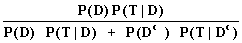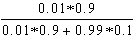=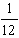2.85 P = Positive Response, M = Male respondent, F = Female respondent. Hence:
P(M) = ¼, P(F) = 3/4, P(P | F) = .7, P(P | M) = .4. Therefore

P(M | Pc) =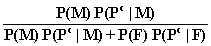=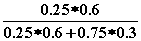= .4

2.90 F = Failure to learn and A and B are the two methods. We are given:
P(A) = 0.3, P(B) = 0.7, P(F | A) = 0.2, P(F | B) = 0.1

P(A | F) =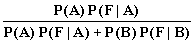= *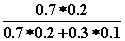= 14/17